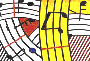Tutorial 35 - Introduction to lambda functions II

Topics

Calling functions randomly using funcall function.

Functions used

funcall, omif, om-random, omloop and om=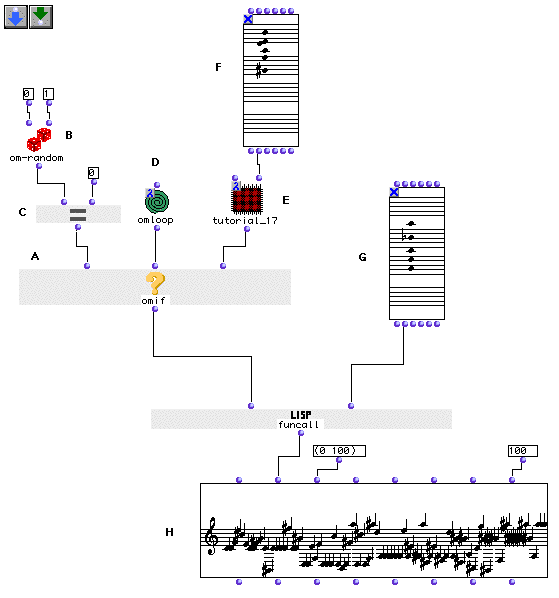Description

We will examine in this patch the possibility to call two different processes in order to transform the same musical material. Starting with a CHORD (G), we will allow a random processing of this chord, using either the process of tutorial 14 which constructs a random sequence of note around some given notes that will be repeated randomly, or the interpolating procedure of tutorial 18.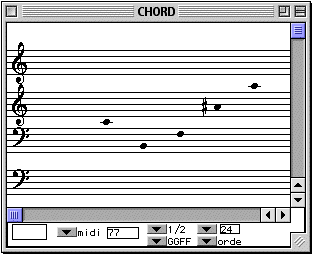CHORD (G), second argument of funcall

This is possible using funcall . funcall takes as a first argument a function. The other arguments are the arguments that is necessary to its function (if the function takes for example 3 arguments, then you must ad to funcall thre extra inputs, c.f funcall function in the OM Reference guide). We will use an omif that will set either function (D) or (E.) One must notice that both functions have one non-conneted input. This input will be the second argument connected to funcall and in this case it is the CHORD (G).

Patch structure

A: omif will be used to set the first argument of funcall following the output of om-random (B)

B: om-random will output either zeros or ones.

C: The om= predicate will check the result of om-random (B) . If the output is zero om= will return 't' . In this case omif (A) will return its second argument, the function (D). If the resulting evaluation of omrandom (B) is 1, om= will return 'nil' and omif (A) will return its third argument which is the function (E).

D: Now let us examine function (D).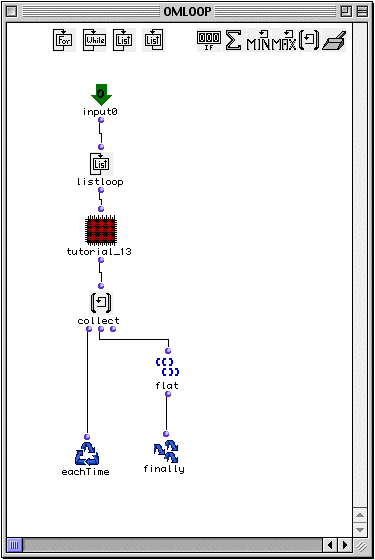In fact this function is an omloop which is in lambda state. Inside the omloop (D) we dragged tutorial_14 and transformed it into a red patch by typing 'a'.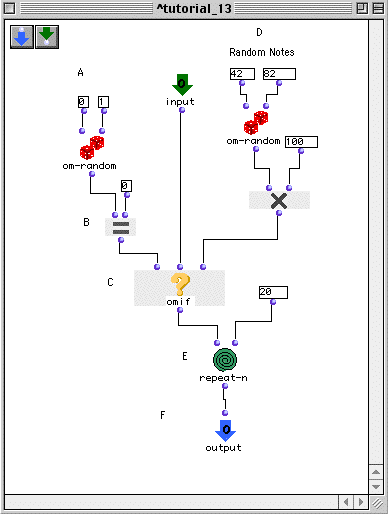We have also added an extra input and an extra output to the patch. The resulting output will be the tutorial_14 process, on each note of the (G) chord.

E: If om= receives 1, omif will return the lambda function (E). This function is again taken from an already existant tutorial (17). We have added extra inputs and one output after we have 'abstracted' the tutorial_18 patch (by typing 'a').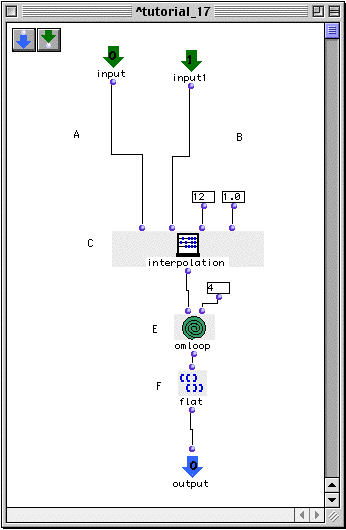As described in tutorial 18, this tutorial will do an interpolation between two chords. This will be done here between the chord (G) (connected to funcall) and the chord (F) which is connected to the second input of tutorial_18.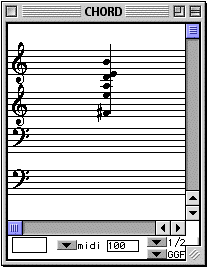Chord F

The output of funcall will therefore be dependent on the omrandom (B) function. One may use conditional and add other transformational procedures in order to have different results. The result shown above is one possible output to our patch.